# Point and line sources/sinks

A number of sources/sinks are available in Porous Flow, implemented as DiracKernels.

## Constant point source

PorousFlowSquarePulsePointSource implements a constant mass point source that adds (removes) fluid at a constant mass flux rate for times between the specified start and end times. If no start and end times are specified, the source (sink) starts at the start of the simulation and continues to act indefinitely. For instance:

[DiracKernels]
[./sink1]
type = PorousFlowSquarePulsePointSource
start_time = 100
end_time = 300
point = '0.5 0.5 0'
mass_flux = -0.1
variable = pp
[../]
[./sink]
type = PorousFlowSquarePulsePointSource
start_time = 600
end_time = 1400
point = '0.5 0.5 0'
mass_flux = -0.1
variable = pp
[../]
[./source]
point = '0.5 0.5 0'
start_time = 1500
mass_flux = 0.2
end_time = 2000
variable = pp
type = PorousFlowSquarePulsePointSource
[../]
[]

(modules/porous_flow/test/tests/dirackernels/squarepulse1.i)

Note that the parameter mass_flux is positive for a source and negative for a sink, which is dissimilar to the conventions used below for line-sink strength and flux .

## PorousFlow polyline sinks in general

Two types of polyline sinks are implemented in PorousFlow: the PorousFlowPolyLineSink and the PorousFlowPeacemanBorehole. These are extensions and specialisations of the general PorousFlowLineSink (that is not available to use in an input file) which is described in this section.

Polyline sinks and sources are modelled as sequences of discrete points: (1) The sink is (2) Here is a volume source, measured in kg.m.s (or J.m.s for heat flow), which when integrated over the finite element yields just the "sink strength", , which has units kg.s for fluid flow, or J.s for heat flow. The sink strength is the parameter that is specified by the user in the input file, and the parameters are convenient weights that are discussed below.

The strength, , is a function of porepressure and/or temperature, and may involve other quantities, as enumerated below. The convention followed is:

• A sink has . This removes fluid or heat from the simulation domain;

• A source has . This adds fluid or heat to the simulation domain.

The input parameters for each PorousFlow polyline sink involve a plain text file whose lines are space-separated quantities: (3)

The weighting terms, , are for user convenience, but for the Peaceman borehole case they are the borehole radius at point .

The basic sink may be multiplied by any or all of the following quantities

• Fluid relative permeability (when use_relative_permeability = true)

• Fluid mobility () (when use_mobility = true)

• Fluid mass fraction (when mass_fraction_component is specified)

• Fluid enthalpy (when use_enthalpy = true)

• Fluid internal energy (when use_internal_energy = true)

That is, in Eq. (2) may be replaced by , , etc. (The units of , , etc, are kg.s for fluid flow, or J.s for heat flow.) All these additional multiplicative factors are evaluated at the nodal positions, not at point , to ensure superior numerical convergence (see upwinding).

Examples of these use_ parameters are provided below. They are usually used in coupled situations, for instance, a fluid sink may extract fluid at a given rate, and therefore in a simulation that includes, the same sink multiplied by fluid enthalpy should be applied to the temperature variable.

warning

When using the PorousFlow Dirac Kernels in conjunction with PorousFlowPorosity that depends on volumetric strain (mechanical = true) you should set strain_at_nearest_qp = true in your GlobalParams block. This ensures the nodal Porosity Material uses the volumetric strain at the Dirac quadpoint(s). Otherwise, a nodal Porosity Material evaluated at node in an element will attempt to use the member of volumetric strain, but volumetric strain will only be of size equal to the number of Dirac points in the element.

## Polyline sinks as functions of porepressure and/or temperature

A PorousFlowPolyLineSink is a special case of the general polyline sink. The function, in Eq. (2) is assumed to be a piecewise linear function of porepressure and/or temperature. In addition, a multiplication by the line-length associated to is also performed. Specifically: (4) where the pre-factor of is the line-length associated to , and (kg.s.m or J.s.m) is a piecewise-linear function, specified by the user in the MOOSE input file (the weights premultiply this as in Eq. (2) before it is used by MOOSE).

For instance:

[DiracKernels]
[./pls]
# This defines a sink that has strength
# f = L(P) * relperm * L_seg
# where
#    L(P) is a piecewise-linear function of porepressure
#      that is zero at pp=0 and 1 at pp=1E7
#    relperm is the relative permeability of the fluid
#    L_seg is the line-segment length associated with
#      the Dirac points defined in the file pls02.bh
type = PorousFlowPolyLineSink

# Because the Variable for this Sink is pp, and pp is associated
# with the fluid-mass conservation equation, this sink is extracting
# fluid mass (and not heat energy or something else)
variable = pp

# The following specfies that the total fluid mass coming out of
# the porespace via this sink in this timestep should be recorded
# in the pls_total_outflow_mass UserObject
SumQuantityUO = pls_total_outflow_mass

# The following file defines the polyline geometry
# which is just two points in this particular example
point_file = pls02.bh

# Now define the piecewise-linear function, L

# First, we want L to be a function of porepressure (and not
# temperature or something else).  The following means that
# p_or_t_vals should be intepreted by MOOSE as the zeroth-phase
# porepressure
function_of = pressure
fluid_phase = 0

# Second, define the piecewise-linear function, L
# The following means
#    flux=0 when pp=0  (and also pp<0)
#    flux=1 when pp=1E7  (and also pp>1E7)
#    flux=linearly intepolated between pp=0 and pp=1E7
# When flux>0 this means a sink, while flux<0 means a source
p_or_t_vals = '0 1E7'
fluxes = '0 1'

# Finally, in this case we want to always multiply
# L by the fluid mobility (of the zeroth phase) and
# use that in the sink strength instead of the bare L
# computed above
use_mobility = true
[../]
[]

(modules/porous_flow/test/tests/dirackernels/pls02.i)

The PorousFlowPolylineSink is always accompanied by a PorousFlowSumQuantity UserObject and often by a PorousFlowPlotQuantity Postprocessor

[UserObjects]
[./pls_total_outflow_mass]
type = PorousFlowSumQuantity
[../]

(modules/porous_flow/test/tests/dirackernels/pls02.i)
[Postprocessors]
[./pls_report]
type = PorousFlowPlotQuantity
uo = pls_total_outflow_mass
[../]

(modules/porous_flow/test/tests/dirackernels/pls02.i)

These types of sinks are useful in describing groundwater-surface water interactions via streams and swamps. Often a riverbed conductance, measured in kg.Pa.s is defined, which is (5) Here is the vertical component of the permeability tensor, is the fluid density, is the fluid viscosity, and is a distance variable related to the riverbed thickness. The other parameters are and , which are, respectively, the length and width of the segment of river that the point is representing. The multiplication by is already handled by Eq. (4), and the other terms of will enter into the piecewise linear function, . Three standard types of are used in groundwater models.

• A perennial stream, where fluid can seep from the porespace to the stream, and vice versa. Then , where involves the river stage height;

• An ephemral stream, where fluid can only seep from the porespace to the stream, but not viceversa has if , and zero otherwise. This is a pure sink since always;

• A rate-limited stream, where fluid can exchange between the groundwater and stream, but the rate is limited. This can be modelled by using a piecewise linear that does not exceed given limits (viz, use one of the above cases, but define the p_or_t_vals and fluxes to limit the fluxes).

## Peaceman Boreholes

Wellbores are implemented in PorousFlowPeacemanBorehole using the method first described by Peaceman (1983). Here is a special function (measured in kg.s in standard units) defined in terms of the pressure at a point at the wall of the wellbore.

### The wellbore pressure

The wellbore pressure is an input into Peaceman's formula. For any along the wellbore, the wellbore pressure is defined as (6) Here

• is an input parameter, bottom_p_or_t. It is the pressure at the bottom of the wellbore.

• is the position (a point in 3D) of the bottom of the wellbore. It is defined to be the last point in the point_file.

• is a weight vector pointing downwards (product of fluid density and gravity), unit_weight. This means that will be the pressure at point in the wellbore, due to gravitational head. If these gravitational effects are undesirable, the user may simply specify (unit_weight = '0 0 0').

Although unusual, PorousFlow also allows to represent a temperature, by setting function_of = temperature, which is useful for non-fluid models that contain polyline sources/sinks of heat.

### Peaceman's fluid flux

Peaceman writes as (7) Let us discuss each term on the RHS separately. For boreholes that involve heat only (with function_of = temperature) the in the above expression and the discussion below should be replaced by the temperature.

### The mobility

Eq. (7) contains , , and , which are the fluid relative permeability, density and viscosity. Hence the term is the mobility, so users should choose to multiply by the mobility (use_mobility = true) when using PorousFlowPeacemanBorehole. Recall that all the multiplicative factors, including mobility, are evaluated at the nodal positions, not the position . This ensures superior numerical convergence.

note

You should almost always set use_mobility=true. The exceptions are when using the volumetric version of PorousFlow (when multiply_by_density = false appears in your input file) or in non-fluid simulations (function_of = temperature).

### The character

The in Eq. (7) is called the character of the wellbore. There are two standard choices (note that depends only on the absolute value ).

• With character = 1 then for , and zero otherwise. In this case the wellbore is called a production wellbore, and it acts as a sink, removing fluid from the porespace.

• With character=-1, for , and zero otherwise. In this case the wellbore is called an injection wellbore, and it acts as a source, adding fluid to the porespace.

Generalising, character may be chosen to be different from , which is useful for time-varying borehole strengths. The above two choices are generalised to:

• if the wellbore is active only for (and has zero strength otherwise);

• if the wellbore is active only for .

### The well constant

The function is called the well_constant of the wellbore, and is measured in units of length. Usually it is computed automatically by PorousFlow using Peaceman's formulae, below.

Peaceman described the form of by performing 2D analytical and numerical calculations to obtain the following formula

(8)

In this formula:

• the borehole is oriented along the direction (this is generalised in PorousFlow: the direction is defined by the plaintext file of points mentioned in Eq. (3));

• and are the diagonal components of the permeability tensor in the plane (defined by a PorousFlow Material, and generalised in PorousFlow to consider the components normal to the borehole direction);

• is the length of the borehole (defined by the plaintext file of points in Eq. (3));

• is the borehole radius (an input parameter, which is encoded in the plaintext file of points as the first entry in each row: see Eq. (3));

• is the effective borehole radius.

For a cell-centred finite-difference approach, Peaceman (1983) found that (9) Here and are the finite-difference spatial sizes.

Other authors have generalised Peaceman's approach to writing for different geometrical situations. Some of these are contained in Chen and Zhang (2009), where they show that for a finite element situation with square elements of size , the borehole at a nodal position, and isotropic permeability

(10)

Note that the 0.113 is substantially different to Peaceman's 0.2, demonstrating that this method of introducing wellbores dependent on the geometry. The user may specify this quantity via the re_constant input parameter (which defaults to Peaceman's 0.28).

### Simple examples

In the following examples, a vertical borehole is placed through the centre of a single element, and fluid flow to the borehole as a function of porepressure is measured. The borehole geometry is

# fully-saturated
# production
[Mesh]
type = GeneratedMesh
dim = 3
nx = 1
ny = 1
nz = 1
xmin = -1
xmax = 1
ymin = -1
ymax = 1
zmin = -1
zmax = 1
[]

[GlobalParams]
PorousFlowDictator = dictator
[]

[Variables]
[./pp]
initial_condition = 1E7
[../]
[]

[Kernels]
[./mass0]
type = PorousFlowMassTimeDerivative
fluid_component = 0
variable = pp
[../]
[]

[UserObjects]
[./borehole_total_outflow_mass]
type = PorousFlowSumQuantity
[../]
[./dictator]
type = PorousFlowDictator
porous_flow_vars = 'pp'
number_fluid_phases = 1
number_fluid_components = 1
[../]
[./pc]
type = PorousFlowCapillaryPressureVG
m = 0.5
alpha = 1e-7
[../]
[]

[Modules]
[./FluidProperties]
[./simple_fluid]
type = SimpleFluidProperties
bulk_modulus = 2e9
viscosity = 1e-3
density0 = 1000
thermal_expansion = 0
[../]
[../]
[]

[Materials]
[./temperature]
type = PorousFlowTemperature
[../]
[./ppss]
type = PorousFlow1PhaseP
porepressure = pp
capillary_pressure = pc
[../]
[./ppss_qp]
type = PorousFlow1PhaseP
at_nodes = false
porepressure = pp
capillary_pressure = pc
[../]
[./massfrac]
type = PorousFlowMassFraction
[../]
[./simple_fluid]
type = PorousFlowSingleComponentFluid
fp = simple_fluid
phase = 0
[../]
[./porosity]
type = PorousFlowPorosityConst
porosity = 0.1
[../]
[./permeability]
type = PorousFlowPermeabilityConst
permeability = '1E-12 0 0 0 1E-12 0 0 0 1E-12'
[../]
[./relperm]
type = PorousFlowRelativePermeabilityCorey
at_nodes = true
n = 2
phase = 0
[../]
[]

[DiracKernels]
[./bh]
type = PorousFlowPeacemanBorehole

# Because the Variable for this Sink is pp, and pp is associated
# with the fluid-mass conservation equation, this sink is extracting
# fluid mass (and not heat energy or something else)
variable = pp

# The following specfies that the total fluid mass coming out of
# the porespace via this sink in this timestep should be recorded
# in the pls_total_outflow_mass UserObject
SumQuantityUO = borehole_total_outflow_mass

# The following file defines the polyline geometry
# which is just two points in this particular example
point_file = bh02.bh

# First, we want Peacemans f to be a function of porepressure (and not
# temperature or something else).  So bottom_p_or_t is actually porepressure
function_of = pressure
fluid_phase = 0

# The bottomhole pressure
bottom_p_or_t = 0

# In this example there is no increase of the wellbore pressure
# due to gravity:
unit_weight = '0 0 0'

# PeacemanBoreholes should almost always have use_mobility = true
use_mobility = true

# This is a production wellbore (a sink of fluid that removes fluid from porespace)
character = 1
[../]
[]

[Postprocessors]
[./bh_report]
type = PorousFlowPlotQuantity
uo = borehole_total_outflow_mass
[../]
[./fluid_mass0]
type = PorousFlowFluidMass
execute_on = timestep_begin
[../]

[./fluid_mass1]
type = PorousFlowFluidMass
execute_on = timestep_end
[../]

[./zmass_error]
type = FunctionValuePostprocessor
function = mass_bal_fcn
execute_on = timestep_end
[../]

[./p0]
type = PointValue
variable = pp
point = '0 0 0'
execute_on = timestep_end
[../]
[]

[Functions]
[./mass_bal_fcn]
type = ParsedFunction
value = abs((a-c+d)/2/(a+c))
vars = 'a c d'
vals = 'fluid_mass1 fluid_mass0 bh_report'
[../]
[]

[Preconditioning]
[./usual]
type = SMP
full = true
petsc_options = '-snes_converged_reason'
petsc_options_iname = '-ksp_type -pc_type -snes_atol -snes_rtol -snes_max_it -ksp_max_it'
petsc_options_value = 'bcgs bjacobi 1E-10 1E-10 10000 30'
[../]
[]

[Executioner]
type = Transient
end_time = 0.5
dt = 1E-2
solve_type = NEWTON
[]

[Outputs]
file_base = bh02
exodus = false
csv = true
execute_on = timestep_end
[]

(modules/porous_flow/test/tests/dirackernels/bh02.i)

meaning that the borehole radius is 0.1m and it is 1m long.

These examples are part of the MOOSE test suite, and they are testing that Eq. (7) is encoded correctly. The parameters common to each of these tests are:

Table 1: Common parameters in the tests

ParameterValue
Borehole radius, 0.1 m
Bottomhole pressure, 0
Gravity0
Unit fluid weight0
Element sizem
Isotropoic permeabilitym
Fluid reference density1000 kg.m
Fluid bulk modulus2 GPa
Fluid viscosityPa.s
Van Genuchten Pa
Van Genuchten 0.8
Residual saturation0
FLAC relperm 2

It is remotely possible that the MOOSE implementation applies the borehole flux incorrectly, but records it as a Postprocessor correctly as specified by Eq. (7). Therefore, these simulations also record the fluid mass and mass-balance error in order to check that the fluid mass is indeed being correctly changed by the borehole.

A production wellbore:

[DiracKernels]
[./bh]
type = PorousFlowPeacemanBorehole

# Because the Variable for this Sink is pp, and pp is associated
# with the fluid-mass conservation equation, this sink is extracting
# fluid mass (and not heat energy or something else)
variable = pp

# The following specfies that the total fluid mass coming out of
# the porespace via this sink in this timestep should be recorded
# in the pls_total_outflow_mass UserObject
SumQuantityUO = borehole_total_outflow_mass

# The following file defines the polyline geometry
# which is just two points in this particular example
point_file = bh02.bh

# First, we want Peacemans f to be a function of porepressure (and not
# temperature or something else).  So bottom_p_or_t is actually porepressure
function_of = pressure
fluid_phase = 0

# The bottomhole pressure
bottom_p_or_t = 0

# In this example there is no increase of the wellbore pressure
# due to gravity:
unit_weight = '0 0 0'

# PeacemanBoreholes should almost always have use_mobility = true
use_mobility = true

# This is a production wellbore (a sink of fluid that removes fluid from porespace)
character = 1
[../]
[]

(modules/porous_flow/test/tests/dirackernels/bh02.i)

yields the correct result (Figure 1 and Figure 2):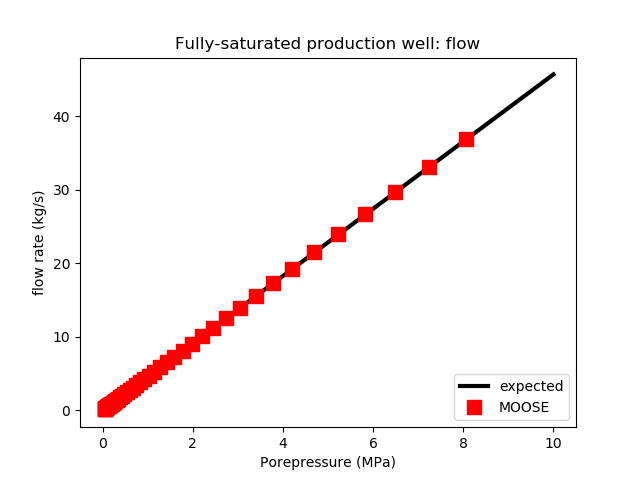Figure 1: The flow to a production wellbore in MOOSE agrees with the expected result.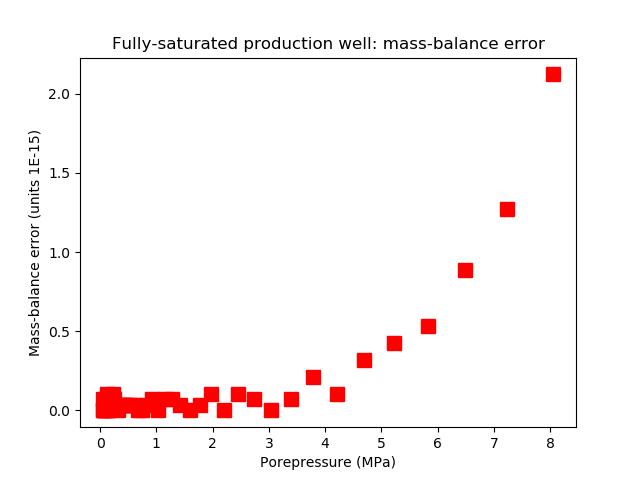Figure 2: The mass-balance error is virtually zero.

An injection wellbore:

[DiracKernels]
[./bh]
type = PorousFlowPeacemanBorehole
variable = pp
SumQuantityUO = borehole_total_outflow_mass
point_file = bh03.bh
function_of = pressure
fluid_phase = 0
bottom_p_or_t = 1E7
unit_weight = '0 0 0'
use_mobility = true
character = -1
[../]
[]

(modules/porous_flow/test/tests/dirackernels/bh03.i)

yields the correct result (Figure 3 and Figure 4):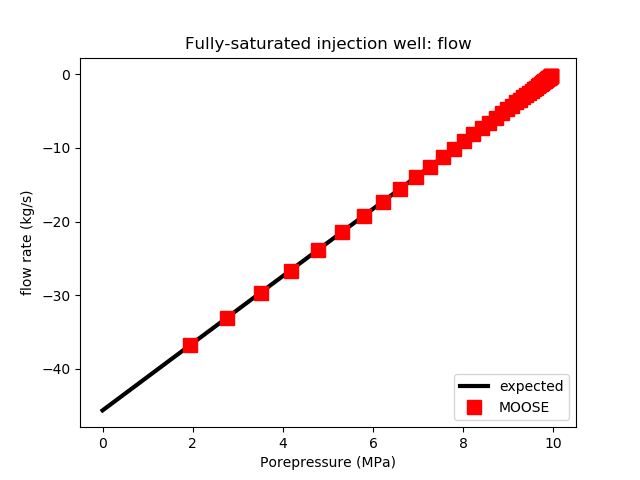Figure 3: The flow from an injection wellbore in MOOSE agrees with the expected result.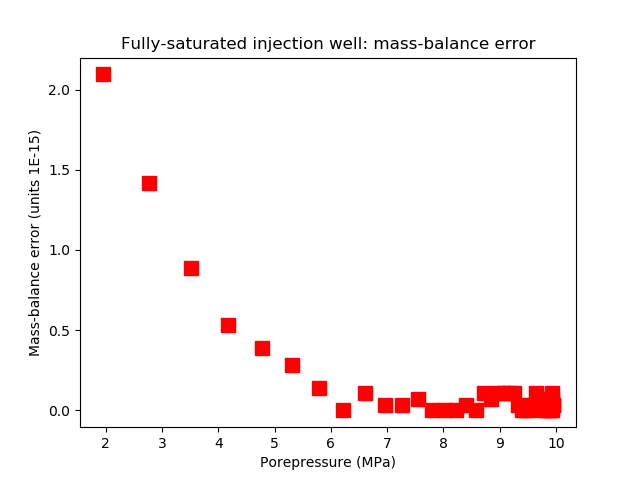Figure 4: The mass-balance error is virtually zero.

A production wellbore with bottomhole porepressure MPa so it forces desaturation:

[DiracKernels]
[./bh]
type = PorousFlowPeacemanBorehole
variable = pp
SumQuantityUO = borehole_total_outflow_mass
point_file = bh02.bh
fluid_phase = 0
bottom_p_or_t = -1E6
unit_weight = '0 0 0'
use_mobility = true
character = 1
[../]
[]

(modules/porous_flow/test/tests/dirackernels/bh04.i)

yields the correct result (Figure 5 and Figure 6):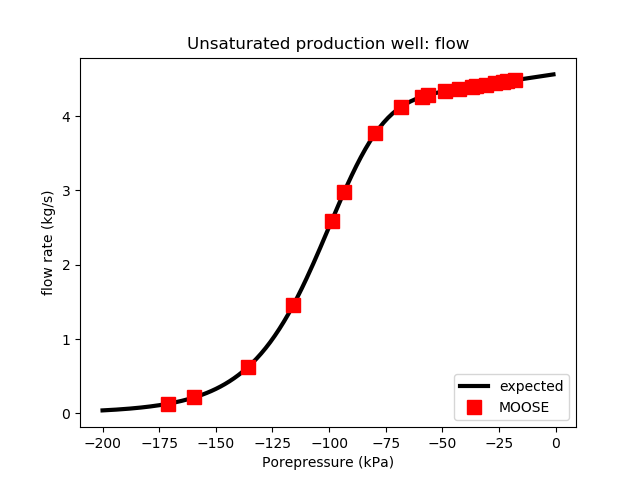Figure 5: The flow to a production wellbore in MOOSE agrees with the expected result.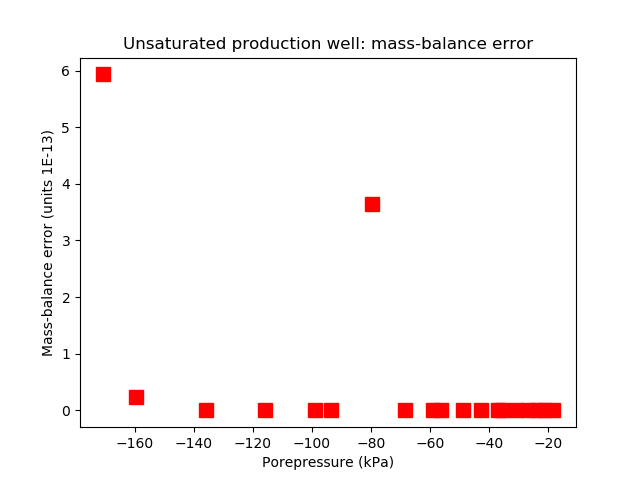Figure 6: The mass-balance error is virtually zero.

An injection wellbore with so it is only active when the rock porepressure is negative:

[DiracKernels]
[./bh]
type = PorousFlowPeacemanBorehole
variable = pp
SumQuantityUO = borehole_total_outflow_mass
point_file = bh03.bh
fluid_phase = 0
bottom_p_or_t = 0
unit_weight = '0 0 0'
use_mobility = true
character = -1
[../]
[]

(modules/porous_flow/test/tests/dirackernels/bh05.i)

yields the correct result (Figure 7 and Figure 8):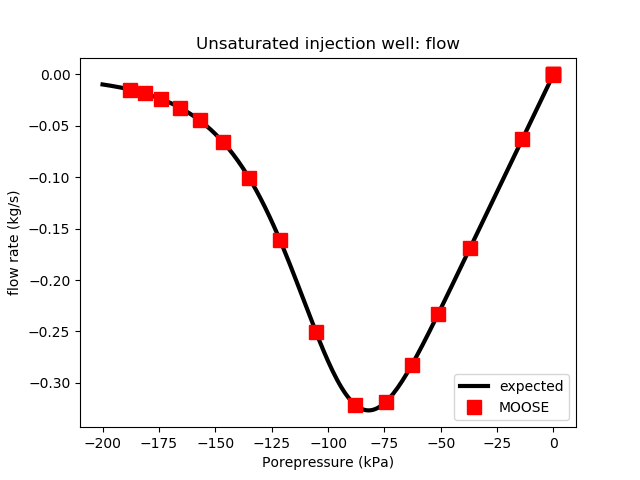Figure 7: The flow from an injection wellbore in MOOSE agrees with the expected result.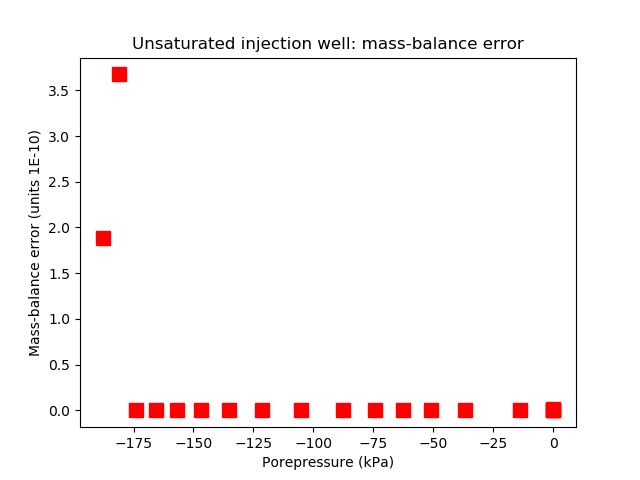Figure 8: The mass-balance error is virtually zero.

Each of these record the total fluid flux (kg) injected by or produced by the borehole in a PorousFlowSumQuantity UserObject and outputs this result using a PorousFlowPlotQuantity Postprocessor:

[UserObjects]
[./borehole_total_outflow_mass]
type = PorousFlowSumQuantity
[../]

(modules/porous_flow/test/tests/dirackernels/bh02.i)
[Postprocessors]
[./bh_report]
type = PorousFlowPlotQuantity
uo = borehole_total_outflow_mass
[../]

(modules/porous_flow/test/tests/dirackernels/bh02.i)

### Reproducing the steady-state 2D analytical solution

The PorousFlow fluid equation (see governing equations) for a fully-saturated medium with and large constant bulk modulus becomes Darcy's equation (11) where , with notation described in the nomenclature. In the isotropic case (where ), the steadystate equation is just Laplace's equation (12)

Place a borehole of radius and infinite length oriented along the axis. Then the situation becomes 2D and can be solved in cylindrical coordinates, with and independent of . If the pressure at the borehole wall is , then the fluid density is . Assume that at the fluid pressure is held fixed at , or equivalently the density is held fixed at . Then the solution of Laplace's equation is well-known to be (13) This is the fundamental solution used by Peaceman and others to derive expressions for by comparing with numerical expressions resulting from Eq. (7).

Chen and Zhang Chen and Zhang (2009) have derived an expression for in the case where this borehole is placed at a node in a square mesh. This test compares the MOOSE steadystate solution with a single borehole with defined by Chen and Zhang's formula (specifically, the re_constant needs to be changed from its default value) is compared with Eq. (13) to illustrate that the MOOSE implementation of a borehole is correct.

The PorousFlowPeacemanBorehole is:

[DiracKernels]
[./bh]
type = PorousFlowPeacemanBorehole
variable = pp
SumQuantityUO = borehole_total_outflow_mass
point_file = bh07.bh
fluid_phase = 0
bottom_p_or_t = 0
unit_weight = '0 0 0'
use_mobility = true
re_constant = 0.1594 # use Chen and Zhang version
character = 2 # double the strength because bh07.bh only fills half the mesh
[../]
[]

(modules/porous_flow/test/tests/dirackernels/bh07.i)

The mesh used is shown in Figure 9.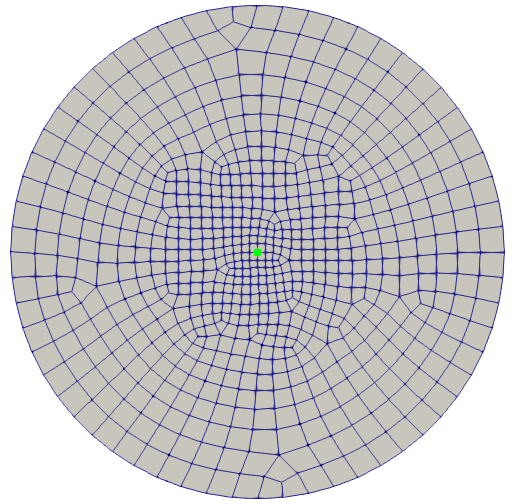Figure 9: The mesh used in the comparison with Eq. (13), with the green dot indicating the position of the borehole. The central elements are m, and the outer boundary is at radius 300m.

Figure 10 show the comparison between the MOOSE result and Eq. (13). Most parameters in this study are identical to those given in the Table 1 with the following exceptions: the mesh is shown in Figure 9; the permeability is m; the borehole radius is 1 m; the borehole pressure is ; the outer radius is m; and the outer pressure is MPa.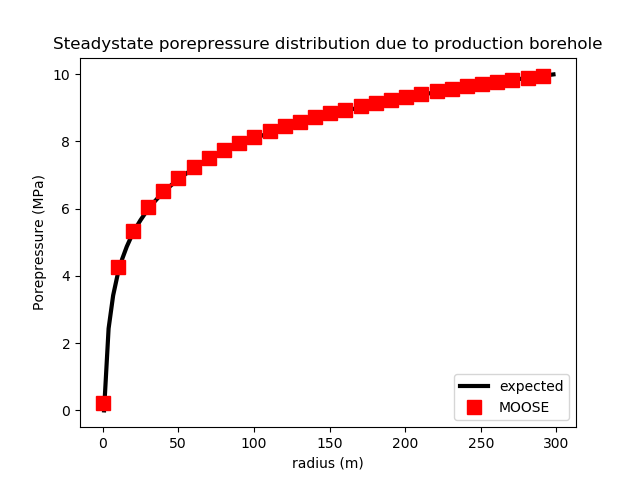Figure 10: Comparison of the MOOSE results (dots) with the analytical solution Eq. (13) for the steadystate porepressure distribution surrounding single borehole.

### Injecting and producing in thermo-hydro simulations

The PorousFlowPeacemanBorehole may be used to model injection and production in thermo-hydro simulations. Suppose that cool water is being injected into an initially hot reservoir via a vertical injection well, and that water is being removed from the system via a vertical production well. In this example we shall study an essentially 2D situation without gravity with parameters defined in Table 2

Table 2: Injection-production parameters

ParameterValue
Injection borehole radius0.2 m
Injection borehole vertical length10 m
Injection bottomhole pressure21 MPa
Injection temperature300 K
Production borehole radius0.25 m
Production borehole vertical length10 m
Production bottomhole pressure20 MPa
Injection-production well separation30 m
Reservoir initial porepressure20 MPa
Reservoir initial temperature400 K
Gravity0
Unit fluid weight0

The fluid properties are defined as:

[Modules]
[./FluidProperties]
[./the_simple_fluid]
type = SimpleFluidProperties
thermal_expansion = 2E-4
bulk_modulus = 2E9
viscosity = 1E-3
density0 = 1000
cv = 4000.0
cp = 4000.0
[../]
[../]
[]

(modules/porous_flow/test/tests/dirackernels/injection_production.i)

The fluid injection and production is implemented in a way that is now familiar:

  [./fluid_injection]
type = PorousFlowPeacemanBorehole
variable = porepressure
SumQuantityUO = injected_mass
point_file = injection.bh
function_of = pressure
fluid_phase = 0
bottom_p_or_t = 21E6
unit_weight = '0 0 0'
use_mobility = true
character = -1
[../]
[./fluid_production]
type = PorousFlowPeacemanBorehole
variable = porepressure
SumQuantityUO = produced_mass
point_file = production.bh
function_of = pressure
fluid_phase = 0
bottom_p_or_t = 20E6
unit_weight = '0 0 0'
use_mobility = true
character = 1
[../]

(modules/porous_flow/test/tests/dirackernels/injection_production.i)

The injection temperature is set via:

[BCs]
[./injection_temperature]
type = DirichletBC
variable = temperature
value = 300
boundary = central_nodes
[../]
[]

(modules/porous_flow/test/tests/dirackernels/injection_production.i)

Alternatively, the heat energy of the injected fluid could be worked out and injected into the heat-conservation equation via a borehole.

The production of fluid means that heat energy must be removed at exactly the rate associated with the fluid-mass removal. This is implemented by using an identical PorousFlowPeacemanBorehole to the fluid production situation but associating it with the temperature variable and with use_enthalpy = true:

  [./remove_heat_at_production_well]
type = PorousFlowPeacemanBorehole
variable = temperature
SumQuantityUO = produced_heat
point_file = production.bh
function_of = pressure
fluid_phase = 0
bottom_p_or_t = 20E6
unit_weight = '0 0 0'
use_mobility = true
use_enthalpy = true
character = 1
[../]

(modules/porous_flow/test/tests/dirackernels/injection_production.i)

Here the heat energy per timestep is saved into a PorousFlowSumQuantity UserObject which may be extracted using a PorousFlowPlotQuantity Postprocessor, which would be an important quantity in a geothermal application:

[Postprocessors]
[./heat_joules_extracted_this_timestep]
type = PorousFlowPlotQuantity
uo = produced_heat
[../]
[]

(modules/porous_flow/test/tests/dirackernels/injection_production.i)

Scaling of the variables ensures good convergence:

[Variables]
[./porepressure]
initial_condition = 20E6
[../]
[./temperature]
initial_condition = 400
scaling = 1E-6 # fluid enthalpy is roughly 1E6
[../]
[]

(modules/porous_flow/test/tests/dirackernels/injection_production.i)

Some results (run on a fine mesh) are shown in Figure 11 and Figure 12.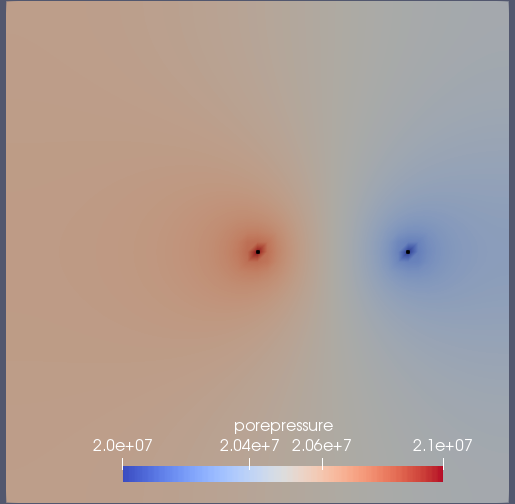Figure 11: Porepressure after s of injection and production. The black spots indicate the wells.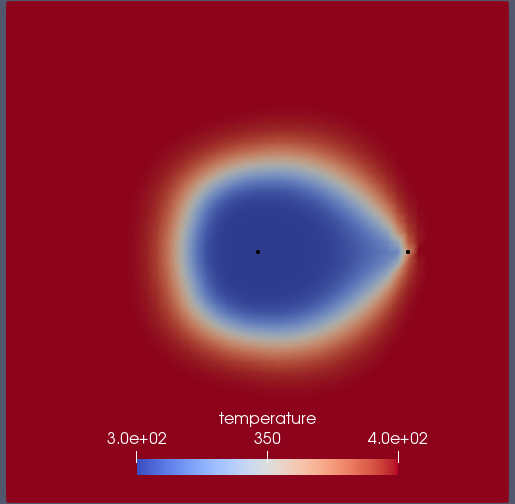Figure 12: Temperature after s of injection and production. the black spots indicate the wells.

1. Z. Chen and Y. Zhang. Well flow models for various numerical methods. International Journal of Numerical Analysis and Modelling, 6:375–388, 2009.[BibTeX]
2. D. W. Peaceman. Interpretation of well-block pressures in numerical reservoir simulation with nonsquare grid blocks and anisotropic permeability. SPE J., 23:531–543, 1983.[BibTeX]